Courses

# Surveying (Part - 14) Civil Engineering (CE) Notes | EduRev

## Civil Engineering (CE) : Surveying (Part - 14) Civil Engineering (CE) Notes | EduRev

The document Surveying (Part - 14) Civil Engineering (CE) Notes | EduRev is a part of the Civil Engineering (CE) Course Civil Engineering SSC JE (Technical).
All you need of Civil Engineering (CE) at this link: Civil Engineering (CE)

Surveying (Part 14)

ELECTRONIC DISTANCE MEASUREMENT (E.D.H)
Electonic distance measuring instrument or electromagnetic distance measuring instrument (called E.D.M. instrument) have been recently developed which give a very high accuracy in distance measurement. An accuracy of 1 in 105 can be achieved  without much difficulty for sights upto 100 k. These methods are extremely useful for indirect measurement of distance over difficult terrains and where a high accuracy is erquired, e.g. base line measurement in triangulation, trilateration and precies traverse.

• The electonics distance measuring instruments can be broad classified into 3-types
1. Light waves instruments
2. Infra-red waves instruments
3. Micro waves instruments The basic theory of all the three types is eassentially the same Electromagnetic waves
• The electromagnetic waves require no medium, and they can travel even in vaccum
• The velocity of light in vaccum = 3×108 n/s
• The ratio of the velocity of electromagnetic waves in vaccum to that in atmosphere is called the refractive index ratio (n)
Thus  n=C/V
or V=C/n

The value of the refractive index ratio (n) is greater than unity. It depends on air temperature, atmospheric condition and relative humidity.
The quantity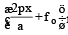is called the phase angle or simply phase of the motion.
At point B phase angle is 90º At point c, 180º and at D = 270º, at E phase angle is 360º. As the cycle repeates after E, the phase angle at E may not be taken as 0º.
The distance travelled by the wave corresponding to different values of the phase angles are :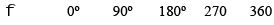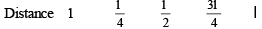As the cycle repreated after f = 360º, the total distance travelled can be represented as where D = na + d n = number of completed waves d = part of the wave The port of the wave d can be written in terms of the wave length (l) if the phase angle f is known.
Because angle of 360º corresponding to one year length (l)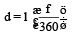Thus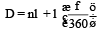Units

• The wave length is measured in metres (m)
• The frequency is measured in hertz (Hz) which corresponds to a frequency of 1 cycle per second.
• The unit of time is second (s).

(c) If n is the number of observations of an angle and V is the residual error i.e. differences between the mean observed value of the angle and its observation value, then weigth of the observation of the angle is given by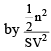This rule is known as Gauss’s rule.

Figure adjustment Determination of the most probable value of the angles of any geometrical figure into fulfill the geometerical conditions. is known as the figure adjustments. The geometrical figures generally used in a triangulation system are the following.
(i) Triangles
(iii) centred polygons

Triangle adjustment: The distribution a triangular error may be mae by one of the following rules:

(a) If the angles of the triangles are of equal wights, then distribute the triangular error equally among the three angles

(b) If the angles of the triangle are of unequal weight distribute, then the triangular error among the three angles inversely to their respective weights.

Correction of angle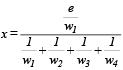Correction of angle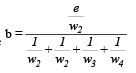Correction of angle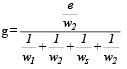Correction of angle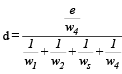(iii) Part horizon having individual and combined angles.

The probable error of a quantity which os a function of a observed quantity is obtained by multiplying the probable error of the observed quantity by the differentiation of the quantity with respect to the observed quantity.
Let x = observed quantity with ex p.e.
y = observed quantity with ey p.e.
and y = f (x)
then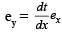(E) The probable error of the quantity which as a function of more than two observed quantities is equal to the square of the summation of the squares of the probable errors of observed quantity multiplied by its differentiation with respect to quantity.
Let x1, x2, x3 -------- xn be the observed quantities
y = computed quantity
y = f (x1, x2, x3.......xn) Then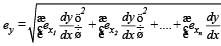whose ex1 , ex2 , ex..... exx are the problem error of the  observed quantities and ey is the probable error of the computed quantity.

Triangulation Adjustment The adjustment of triangulation is generally carried out two steps:

Probable error While determining the probable error of observation the following cases may arise

(A) Direct observation of equal weights
(a) The probable error of single observations of unit weight
(b) The probable error of any observation of wieght,

(c) The probable erro fo arithmetic mean observation
(B) Direct observations of unequal weights
(C) Computed quantities

(A) Direct observations of equal weights

(a) The probable error of single observation weight unit of may be calculated from the following formula.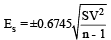where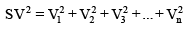V = Residual errors = observed value of the quantity – probable value of the quantity

n = total number of observations

(b) The probable error of any observation of weight, we  may be calculated from the following formula.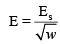Station Adjustment. Depending upon the method of obsevation the following the cases may arise.

(i) A close horizon with angles of equal weights
(ii) A close horizon with angles of unequal weights.
(iii) Part horizon with angles observed individually and also in combination.

(i) Closed horizon with angles of equal weights.
Let a, b, g and d be the observed angles of  To get the most probable values of the angles the error of closure is distributed equally
(ii) Closed horizon with angles of unequal wts.
In this case, the error of closure is distributed among the angles inversely as their weights.
Let e be the closure error

Correction of angle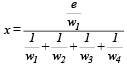Correction of angle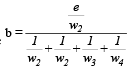Correction of angle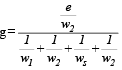(B) Conditioned quantities

When the observation are a combination of observation equations, and conditioned equation the M.P.Vs may be obtained by the following method.

(1) By avoiding the conditioned equation and forming the normal equations of the unknowns.
(2) By avoiding the observation equations and finding the values of unknown by the correlated.

1. Method of Normal equation

2. Method of difference : Let k1, k2, k3 .... be the most probable corrections to the observed angle A, B, C.
The most probable values of A, B and C are A = observed value +k1
B = observed value + k2
C = observed value + k3

so k1, k2, k3 be the residual error \ Residual error = M.P.V. – observed value

(C) Methods of correlates.
Direct observations of unequal weight

(a) The probable error of a single observation of unit wt.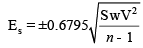(b) The probable error of anyf observation of weight w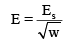(c) The probable error of weighted arithematic mean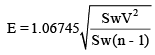where, n = number of observations w = weight of an observation Iw = sum of the weights Iwr2 = sum of the weighted squares of the residual

(3) The computed quantities.

The probable errors of computed quantities may be calculated by the following laws

(a) The probable error of any computed quantities which is either the sum or the difference of the observed quantities plus or minus a constant is always the same as that of observed quantity.
(b) The probable error of the multiple of the observed quantity and a constant is obtained by multiplying the computed quantity by the same constant.
(c) The probable error of the sum of the observed quantities is equal to the square root of the sum of the squares of the probable errors in the observed quantities.

Weight of an observation The relative precision and trust worthiness of an observation as compared to the precision of other quantities is known as weight of the observations. It is always expressd in number. The higher number indicates higher precision as compared to lower number.

Offer running on EduRev: Apply code STAYHOME200 to get INR 200 off on our premium plan EduRev Infinity!

## Civil Engineering SSC JE (Technical)

109 docs|50 tests

,

,

,

,

,

,

,

,

,

,

,

,

,

,

,

,

,

,

,

,

,

;